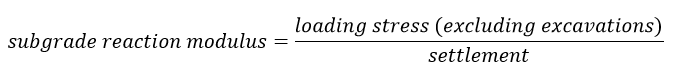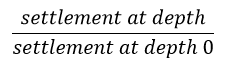RocFall3 | Your new tool for 3D assessment of slopes at risk for rockfalls. Get started with RocFall3 today!

# Modulus of Subgrade Reaction

The Modulus of Subgrade Reaction is the total applied structural load divided by the total resulting settlement. The total applied structural load is considered to be the loading stress calculated from any load other than an excavation.

The subgrade reaction modulus can be calculated using total, consolidation, or immediate settlement.## Minimum settlement ratio for subgrade modulus

The Minimum settlement ratio for subgrade modulus option limits the range of the calculated subgrade reaction modulus values. The settlement ratio is calculated as: